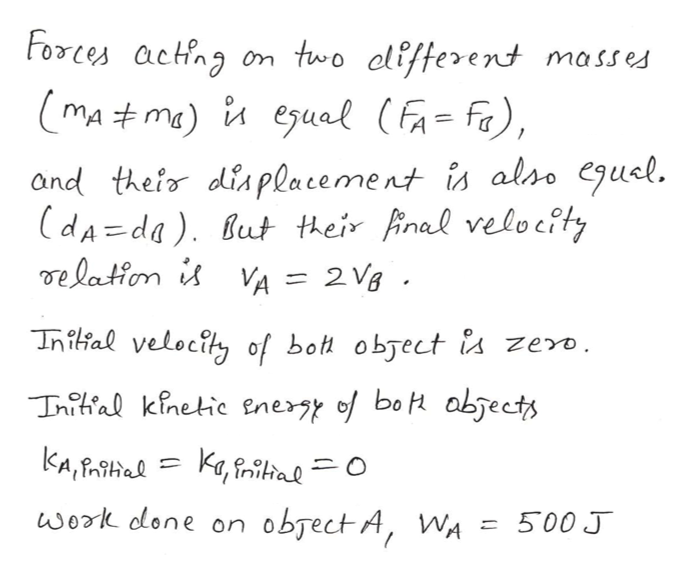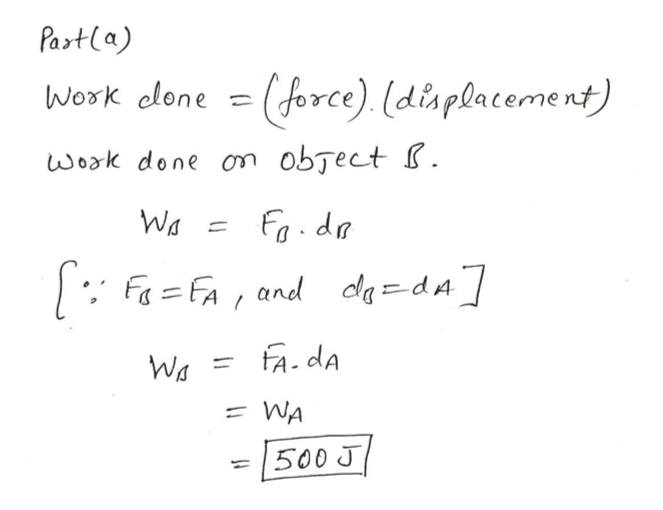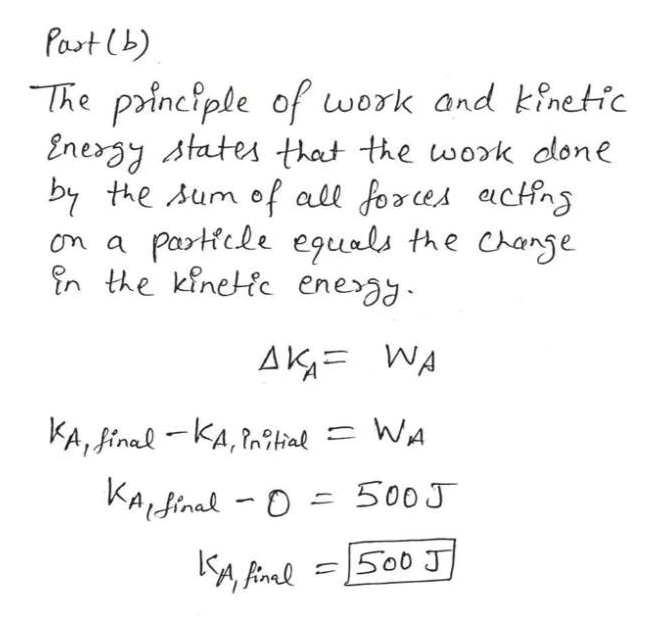# Two objects of different mass start from rest, are pulled by the same magnitude net force, and are moved through the same distance. The work done on object A is 500 J.  After the force has pulled each object, object A moves twice as fast as object B.How much work is done on object B? What is the kinetic energy of object A after being pulled? What is the kinetic energy of object B after being pulled?    What is the ratio of the mass of object A to the mass of object B?

Question

Two objects of different mass start from rest, are pulled by the same magnitude net force, and are moved through the same distance. The work done on object A is 500 J.  After the force has pulled each object, object A moves twice as fast as object B.

How much work is done on object B?

What is the kinetic energy of object A after being pulled?

What is the kinetic energy of object B after being pulled?

What is the ratio of the mass of object A to the mass of object B?

check_circle

Step 1help_outlineImage TranscriptioncloseForces acting two clifferent masses mA#ma) i eual (Fa=fa), and thefor diaplacement is also egusl. (dA=da). But their final velocty de laton is VA = 2 Vg Inilal velocity of bot object a zero. Tnitial kinetic enery of bo abjects KA,Halka,iltal O work done on obsect A, WA 500 J fullscreen
Step 2help_outlineImage TranscriptioncloseParta) Work clone =(force). (displacement) work done on object I. Wa ) =FA, and deda FA.dA Wa WA = |500 J fullscreen
Step 3help_outlineImage TranscriptionclosePast (b) The principle of work and kinetic Energy states that the wok done by the Aum of all forces actfn Partfcle eguals the Change n the kinetic ene am a AK WA KA, inal KA,l WA KAdinal-D 500J S00 J 1 fullscreen

### Want to see the full answer?

See Solution

#### Want to see this answer and more?

Solutions are written by subject experts who are available 24/7. Questions are typically answered within 1 hour.*

See Solution
*Response times may vary by subject and question.
Tagged in

### Work,Power and Energy